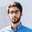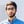# em vs. px in CSSNouman Abbasi

em and px are commonly used length units in CSS. In this shot, we will cover the differences between em and px.

First, let’s try to understand the types of length units in CSS.

## Length units

There are two types of length units:

1. Relative
2. Absolute

Relative length units represent a length relative to another length. Style sheets that use relative units can scale from one output environment to another more easily.

em is an example of a relative length unit.

Absolute length units are fixed about each other and represent an actual physical measurement. They are only recommended when the output environment is known.

px is an absolute unit.

## Differences

The main difference between em and px is their length unit types.

em is font-relative unit ,i.e., em is equal to the computed value of the font-size property of the element on which it is used.

Use em when you specifically want the size of something to depend on the current font size.

On the other hand, px or pixel, is an absolute unit, where $1$ px $= 1/96$th of an inch. However, pixels are relative to the viewing device.

Use px if you want to incorporate fixed-size elements, such as logos and icons. For example, in the case of images, each pixel is 1px in size, so if you are designing around an image, you’ll need px units.

## Code

In this example, we have two paragraphs, one that uses em and the other that uses px as the units for font-size.

• HTML
• CSS (SCSS)

RELATED TAGS

css

CONTRIBUTORNouman Abbasi